## Viscosity

Viscosity is that property of a fluid which is the measure of its resistance to flow (i.e. continual deformation). Viscosity can depend on the type of flow (shear and/or extensional), its duration and rate, as well as the prevailing temperature and pressure. Quantitatively, viscosity is defined as the stress in a particular ideal flow-field divided by the rate of deformation of the flow.
In shear flow—where we imagine the flow as hypothetical layers of fluid flowing over each other—we define the relevant parameters as (see Figure 1) σ the shear stress (force per unit area) at the boundary of the fluid to produce the flow, and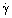the shear rate (sometimes called the strain rate or velocity gradient), which is the proper measure of the rate of deformation in the fluid undergoing shear flow. The ratio of these two quantities is the viscosity; hence η = σ/. The units of shear stress are Pascals (Pa), shear rate reciprocal seconds (s−1), and so the unit of viscosity is the Pascal-seconds (Pas or Pa.s), with mPas being the more usual unit used for low viscosity fluids. (Prior to the introduction of the SI system, the cgs unit relevant to low viscosity liquids was the centipoise, which is identical to the mPas.) A form of viscosity often referred to is the kinematic viscosity, ν m2s−1 which is the quantity we have defined above divided by the density of the fluid, ρ, i.e., ν = η/η.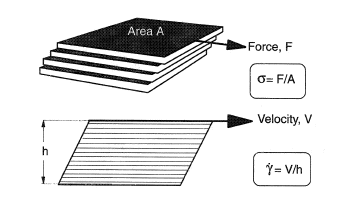Figure 1.
If the viscosity of a fluid does not change with type, time and rate of deformation, we call it a Newtonian Fluid, otherwise it is known as a Non-Newtonian Fluid. The most common Newtonian liquid is water, and this provides us with the international standard for viscosity. All other viscosity standards are derived by comparison with the internationally accepted standard for water — 1.025 mPas at 20°C. The approximate viscosity of some other Newtonian fluids (mostly at room temperature) is shown in Table 1.
Table 1. The approximate viscosity of some Newtonian liquids at room temperature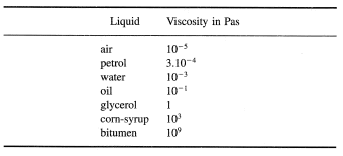The temperature dependence of the viscosity of Newtonian liquids is such that the viscosity decreases with temperature, and in general, the higher the viscosity, the greater the rate of decrease with temperature. Around room temperature, the viscosity of water decreases by 3% per degree Celcius; oils by about 5%, and bitumen by 15% or more.
The viscosity of liquids almost always increases with pressure, with water being the sole exception. Its dependence is such that at the normal pressures found in heat and mass transfer operations, it can usually be neglected.
The most important variation of viscosity for non-Newtonian liquids is with shear rate. The (almost) universal behavior of all such liquids is shown in Figure 2. At low enough shear rates, the viscosity is constant, thereafter decreasing through what is called the power-law region, to eventually flatten out a higher shear rates, often to become constant again, but sometimes rising. The absolute position of this curve on the viscosity and shear rate axes depends on the particular non-Newtonian liquid being investigated, as does the slope of the power-law region, as well as the possible upturn at high shear rate. Depending on the range of shear rates available in Viscosity Measurement different parts of the curve will be accessed for different liquids. If a standard laboratory viscometer is employed, with a shear rate range of typically 1−1000 s−1, then different types of behavior might be seen, as shown in Figure 3. In Figure 3a, the data is plotted linearly as shear stress against shear rate, and transformed in Figure 3b to viscosity versus shear rate plotted on logarithmic axes: from 3b, we can identify which part of the universal curve we are on. From the linear plot 3a, we see that for a number of liquids it looks as if the stress extrapolates to a yield stress. This would be the stress that has to be exceeded before flow begins. This is a useful approximation for the flow rate curve over the shear rate range of measurement only and this in fact was the first-ever non-Newtonian law to be expounded.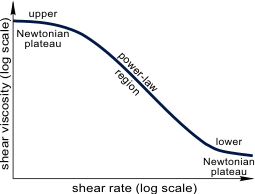Figure 2.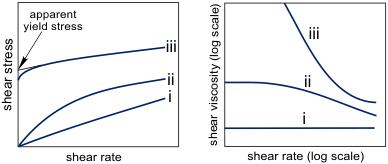Figure 3.
The viscosity of non-Newtonian liquids usually arises from the presence of at least one dispersed phase in a liquid. The deviation of the flow lines caused by the presence of particles of the dispersed phase increases the resistance, i.e., the viscosity. At higher concentrations, hydrodynamic and physical interactions between suspended particles increases the resistance even further. The flow itself can rearrange the spatial order of the particles, so that the random arrangement at low shear rates can become ordered into strings and layers of particles at high shear rates. This results in the lowering of the viscosity with increasing shear rate. It is possible for this ordered arrangement to become disturbed and some degree of three-dimensional structure returns, either as random packing or as clusters. The viscosity in these circumstances increases, and shear thickening takes place—this was previously called by the confusing name of dilatancy. This description of particles generally holds for dispersions of both solid and deformable particles. The increase in viscosity is particularly large for flocculated systems, where the particles attract each other. The decrease in viscosity with increase in shear rate in this case is enhanced by the decrease in size of the flocculated particles.
Qualitatively, the same curve is seen for polymeric systems (see Polymers) but for different reasons. The extended coils viscosify the liquid phase, but overlap—which leads to entanglements— giving the very high viscosities often seen in polymeric solutions. As the shear rate is increased, the coils can distort to become aligned to the direction of flow and coincidentally the number of entanglements decreases—these factors result in the viscosity decreasing with increase in shear rate. At very high shear rates, it is possible to see an increase in viscosity with polymeric systems, but this is rare.
Viscosity can be a function of the time of shearing. As we have explained above, the microstructure of particles and polymer coils can be changed by the imposed flow, however, this takes time. For systems where the change is great and the times needed to affect them are long, the effect is called thixotropy. Just as it takes time to break the structure down, so also it takes time to build it up. This means that the viscosity will decrease towards equilibrium when we increase shear rate, but increase towards equilibrium when we decrease shear rate. This is illustrated in Figure 4, and is seen in its most extreme form for clay-thickened liquids like drilling muds and thixotropic paints. (It is possible for the opposite to happen, especially with shear thickening (dilatant) systems, but this is rare: such behavior is called anti-thixotropy.)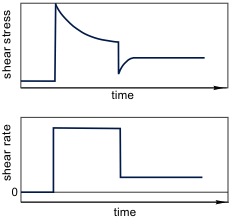Figure 4.
Extensional flow is where, unlike shear flow the velocity gradient is at right angles to the flow, it is along the flow. For applications in real flow geometries, the most usual example is flow into and out of a constriction, as for instance an orifice plate with a small hole. For liquids where the microstructure is alienable, such as linear polymer chains and fibers etc., it is possible for the viscosity appropriate to this flow—the extensional viscosity—can be orders of magnitude higher than in shear flow.
Other forms of viscosity and their form can be found in "An Introduction to Rheology", Barnes, Hutton and Walters, Elsevier, Amsterdam (1989).